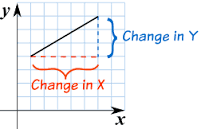# Basic Geometry

Geometry Level 1The line $PQ$ has equation $4x+3y=15$. Find the gradient of $PQ$.

×